Radmanović, K., Roginić, R., Beljo Lučić, R., Jovanović, J., Jug, M., Sedlar, T., and Šafran, B. (2021). "Effect of a high loading rate on the compressive properties of beech wood in the longitudinal direction," BioResources 16(2), 4093-4105.

#### Abstract

Because of its hardness, wear-resistance, strength, and bending capabilities, beech wood is a widely-used hardwood in Europe. It is mainly used for furniture, floors, construction, veneer boards, and laminated wood. For such uses, the mechanical properties are very important, especially in cases of impact loads. The aim of this work is to analyse the mechanical properties of beech wood samples exposed to compressive force in the longitudinal direction based on different loading rates (in the range from 10 mm/min to 500 mm/min). Stress–strain diagrams were made with the experimental data, and mathematical functions were fit to them. Using the fit functions, the following properties of beech wood samples were determined: the stress and strain at the elastic limit; the maximum stress and associated strain; the modulus of elasticity; the tangent modulus; the specific energy of elastic strain; and the specific energy of plastic strain. The results showed that by increasing the loading rates, the elastic properties of beech wood increase, while the analysed plastic properties do not show a clear tendency of changes with increase of the loading rates.

Effect of a High Loading Rate on the Compressive Properties of Beech Wood in the Longitudinal Direction

Kristijan Radmanović,* Robert Roginić, Ružica Beljo Lučić, Juraj Jovanović, Matija Jug, Tomislav Sedlar, and Branimir Šafran

Because of its hardness, wear-resistance, strength, and bending capabilities, beech wood is a widely-used hardwood in Europe. It is mainly used for furniture, floors, construction, veneer boards, and laminated wood. For such uses, the mechanical properties are very important, especially in cases of impact loads. The aim of this work is to analyse the mechanical properties of beech wood samples exposed to compressive force in the longitudinal direction based on different loading rates (in the range from 10 mm/min to 500 mm/min). Stress–strain diagrams were made with the experimental data, and mathematical functions were fit to them. Using the fit functions, the following properties of beech wood samples were determined: the stress and strain at the elastic limit; the maximum stress and associated strain; the modulus of elasticity; the tangent modulus; the specific energy of elastic strain; and the specific energy of plastic strain. The results showed that by increasing the loading rates, the elastic properties of beech wood increase, while the analysed plastic properties do not show a clear tendency of changes with increase of the loading rates.

Keywords: Stress; Strain; Modulus of elasticity

Contact information: University of Zagreb, Faculty of Forestry, Department of Process Engineering, Svetošimunska cesta 25, Zagreb 10000 Croatia; *Corresponding author: kradmanovic@sumfak.hr

INTRODUCTION

Beech wood, due to its mechanical properties, is widely used for the production of furniture and floors. Also, beech wood is suitable for making panels in combination with other materials and is used as construction material. Therefore, in use, beech wood is very often exposed to impact loads, and it is important to know the effects of the loading rates on the compressive properties of beech (Fagus sylvatica) wood. In this paper, the effect of the loading rate on the stress and strain at the elastic limit; the maximum stress and associated strain; the modulus of elasticity; the tangent modulus; the specific energy of elastic strain; and the specific energy of plastic strain of beech wood in the longitudinal direction are determined. The elastic and plastic compressive properties of the beech wood samples were calculated using the simple functions fit to measured data.

EXPERIMENTAL

The experimental research was carried out in two phases. In the first phase, the wood samples were selected and prepared. In the second phase, the compressive properties of the wood samples were measured in the longitudinal direction. The testing of the compressive properties of the wood samples was carried out at the accredited Wood Laboratory for Construction at the Faculty of Forestry and Wood Technology of the University of Zagreb.

Materials

It is known that the mechanical properties of wood depend on the dimensions of the examined samples or on the structure of the wood samples (Horvat and Krpan 1967; Hu et al. 2019).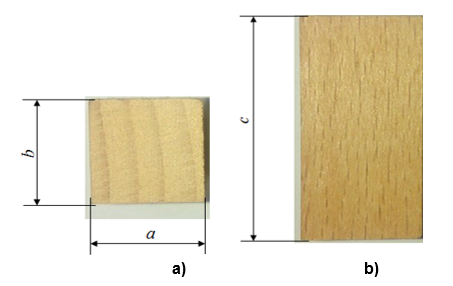Fig. 1. Beech wood samples: a) cross section; and b) radial section (a – length of the beech sample, b – width of the beech sample, and c – height of the beech sample)

To determine as precisely as possible the energy required for the elastic and plastic strain of the unit volume, the aim of this study was to perform compression tests with the dimensions of the samples as small as possible, while still maintaining good quality in terms of testing machine measurements. The selected samples were free of visible defects that could have a major impact on the measured results. All samples were taken from the same log from the region of the north-western Croatia. The dimensions of the samples were a and b equal to 1.5 cm and c equal to 3 cm (Fig. 1), and the resistance hygrometer showed the moisture content was 10%.

Methods

In the second phase of the experiment, the compressive properties of the beech samples were measured via the compression tests in the longitudinal direction. Figure 2 shows the radial section of the samples with dimensions before and after application of the load. The forces and corresponding displacements (Δc = c – c‘) were measured with a Shimadzu testing machine (type Autograph AG-X), which was equipped with a load cell with a nominal force of 100 kN. The flow and test procedures were programmed with the corresponding software (Trapezium X, Shimadzu, Kyoto, Japan). The displacement measurement (Δc) was performed until the force reached its maximum value.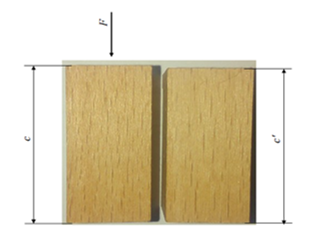Fig. 2. The force action on the beech samples in the longitudinal direction: c is the sample height before action of the force and c‘ is the sample height after action of the force

The compressive properties of the beech wood samples were determined depending on the loading rates, and the selected loading rates were specified as vi.e., 10, 20, 30, 40, 50, 100, 200, 300, 400, and 500 mm/min. During the test, the loading rate was constant. The manufacturer of testing machine states a sufficiently high measurement accuracy of the compressive properties in the measuring range up to 700 mm/min.

The data of the measured force and displacement was processed in SigmaPlot (Version 10.0). The true compressive stress was determined according to Eq. 1,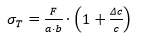(1)

where σis the true stress (Pa), is the force (N), is the sample length (m), b is the sample width (m), c is the initial height of the sample (m), and Δc is the displacement (m). The corresponding strain was determined according to Eq. 2,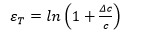(2)

where εT is the true relative strain. After calculating the true compressive stresses and the corresponding true relative strain, the stress-strain diagrams were made. The stress-strain diagram under the compressive action of the force (Fig. 3) can be divided into three parts. In the first part, the strain is smaller than the initial strain (εis less than ε,T0) and the relationship between the stress and strain is nonlinear. The second region is called the elastic region (ε,T0 is less than εT, which is less than εTell), and the relationship between the stress and strain is linear. The point located at the intersection of the second and third parts (Fig. 3) is called the elastic limit (ell), which marks the end of the elastic area and the beginning of the plastic area. In the plastic region (εTell is less than εT, which is less than εTmax); permanent deformations occur, which remain even after the action of force, and the relationship between the stress and strain is nonlinear.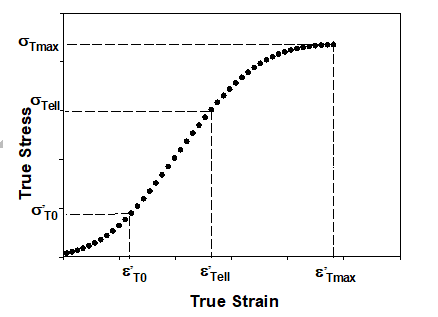Fig. 3. The stress–strain diagram under the action of force in the longitudinal direction. Note: ε´T0 is the true initial strain in the longitudinal direction, ε´Tell is the true strain at the elastic limit in the longitudinal direction, ε´Tmax is the maximum true strain in the longitudinal direction, σ´T0 is the true initial stress in the longitudinal direction, σTell is the true stress at the elastic limit in the longitudinal direction, and σTmax is the maximum true stress in the longitudinal direction

Determination of the elastic properties of beech wood

The elastic properties of beech wood samples determined in this paper are as follows: the modulus of elasticity, the true stress and strain at the elastic limit, and the specific energy of elastic strain. At the beginning of the force action, in addition to compression, the sample was also adjusted on the testing machine due to micro-irregularities on its surface. The strain (ε,T0) (Fig. 3) is the sum of the strain caused by the adjustment of the sample (εT0) and the strain caused by the initial elastic compression of the sample (ε,T0 – εT0). In order to determine the strain caused by the adjustment of the sample (εT0), the experimentally measured data in the linear region of stress-strain diagrams (Požgaj 1993) was fit to a function, as defined by Eq. 3,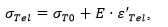(3)

where σTel is any stress less than σTell (Pa), εTel is any strain for which it is valid, i.e.ε,T0 is less than or equal to εTel which is less than or equal to εTellσT0 is the section of a linear function on the ordinate (Pa), and is the modulus of elasticity (Pa). The strain caused by the adjustment of the sample (εT0) was determined by equalizing the linear function (as shown in Eq. 3) with zero, as defined by Eq. 4,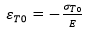(4)

where εT0 is the section of a linear function on the abscissa. Figure 4a shows the stress-strain diagram with an adjusted linear function in the elastic region bounded on the abscissa by strain due to pattern adjustment (εT0) and strain at the elastic limit (ε,Tell), as well as strain on the coordinate axis by stress at the elastic limit (σTell).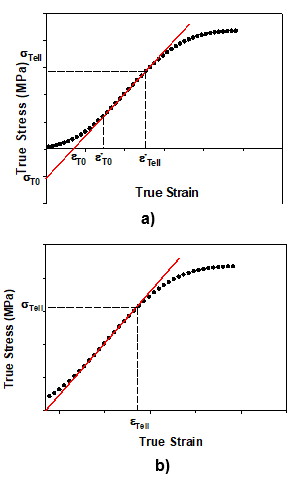Fig. 4. a) The stress–strain diagram under the action of force in the longitudinal direction. Note: σT0 is the section of the linear function on the ordinate, εT0 is the section of a linear function on the abscissa axis, and ε´Tell is the true strain at the elastic limit. b) The translated stress–strain diagram under the action of a force in the longitudinal direction. Note: σTell is the true stress at the elastic limit, and εTell is the true strain at the elastic limit

After determining the strain (εT0), the stress-strain diagram was translated to the left, as shown in Eq. 5,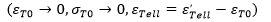(5)

with the aim of eliminating the strain caused by the adjustment of the sample (as shown in Fig. 4b.

After eliminating the strain caused by the adjustment of the sample, the fit function (as shown in Eq. 3) became Eq. 6,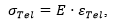(6)

where σTel is any stress less than σTell (Pa), εTel is any strain less than εTell, and is the modulus of elasticity (Pa). The stress at the elastic limit is determined according to Eq. 7,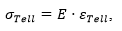(7)

where σTell is the stress at the elastic limit (Pa) and εTell is the strain at the elastic limit. During the process of deformation of the beech samples, the force performs a certain work. One part of the work performed by this force accumulates in the material in the form of potential deformation energy. One part of this energy is the specific energy of the elastic strain, and is determined by Eq. 8,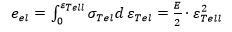(8)

where eel is the specific energy of elastic strain (J/m3), εTell is the strain at the elastic limit, σTel is any stress less than σTell (Pa), εTel is any strain less than εTell, and is the modulus of elasticity (Pa).

Determination of the plastic properties of beech wood

According to Wouts et al. (2016), the stress-strain relationship in the plastic region can be described by a second-order function. The function was fit to the experimentally measured data in the plastic area, as shown in Eq. 9,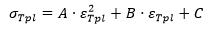(9)

where σTpl is any stress (Pa) for which it is valid, i.e.σTell is less than σTpl which is less than or equal to σTmaxεTpl is any strain for which it is valid, i.e.εTell is less than εTpl which is less than or equal to εTmax, and AB, and C are the coefficients that describe the behaviour of the function of the second order (Pa). Figure 5 shows the plastic region of the stress-strain diagram under the compressive action of a force in the longitudinal direction with the corresponding quadratic function.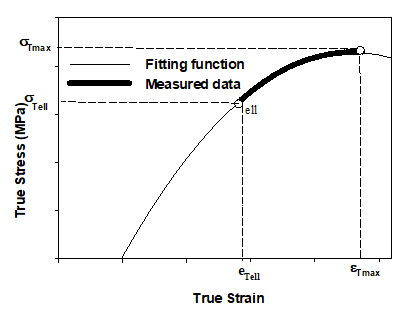Fig. 5. The plastic area of the stress–strain diagram under the action of a force in the longitudinal direction. Note: σTmax is the maximum true stress, σTell is the true stress at the elastic limit, εTell is the true strain at the elastic limit, and εTmax is the maximum true strain

Once the mathematical expressions describing the stress-strain relationship in the elastic and plastic regions have been determined, the strain at the elastic limit is analytically determined by equalizing Eqs. 6 and 9, as shown in Eq. 10,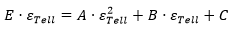(10)

Since two solutions exist for Eq. 10, by comparing them with experimentally measured data, a solution is determined that satisfies the set conditions, as shown in Eq. 11,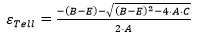(11)

By including Eq. 11 in Eq. 7, the stress at the elastic limit is determined, as shown in Eq.12,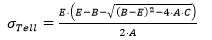(12)

The maximum strain (εTmax) represents the point at which the quadratic function reaches the maximum value, and is determined by equalizing the derivative of Eq. 9 with zero, as shown in Eq. 13,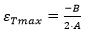(13)

By including Eq. 13 in Eq. 9, the maximum stress (σTmax) is determined, as shown in Eq. 14,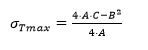(14)

The tangent modulus (K) represents the slope of the tangent to the curve in the plastic region, and is defined as the first derivative of Eq. 9, as shown in Eq. 15,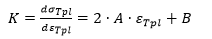(15)

It can be determined from Eq. 15 that the tangent modulus is linearly dependent on the plastic strain, and the linearity coefficient is twice the constant A (2A). The tangent modulus has a maximum value for strain at the elastic limit (εTell), while at its maximum strain (εmax) it is equal to 0.

The mean value of the tangent modulus () in the longitudinal direction, and is determined according to Eq. 16,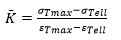(16)

In the process of deformation of the beech samples, an external force performs a certain work that accumulates in the material in the form of potential energy of plastic strain. The elementary strain work invested in the plastic strain of a unit volume is also called the specific energy of plastic strain (epl), and it is determined according to Eq. 17,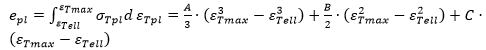(17)

Since the aim of the research was to determine the effect of the loading rate on the compressive properties of beech wood, the experiment was performed in a wide range of loading rates, with five replications under the same conditions. Mathematical functions were fit on measured values for all samples with R2 from 0.995 to 0.999. The mean values of analysed properties with the corresponding standard deviation were determined using fit functions. Due to a small number of the experiment replication, no statistical analysis was done, but the trends of the results are obvious.

RESULTS AND DISCUSSION

Results of Measuring the Elastic Properties of Beech Wood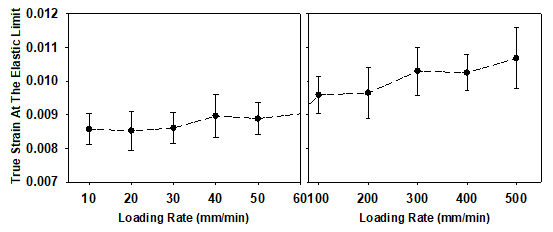Fig. 6. Diagram of the dependence of the true strain at the elastic limit (εTell) on the loading rate under compressive force action in the longitudinal direction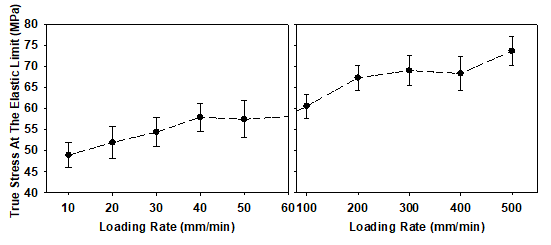Fig. 7. Diagram of the dependence of the true stress at the elastic limit (σTell) on the loading rate under compressive force action in the longitudinal direction

Hu et al. (2019) states that for beech wood samples the stress at the elastic limit under the compressive action of a force in the longitudinal directions is approximately 40 MPa. In the case of the highest loading rate in our experiment the true stress at the elastic limit was almost two times higher. It is likely that the high loading rate is the reason for this difference.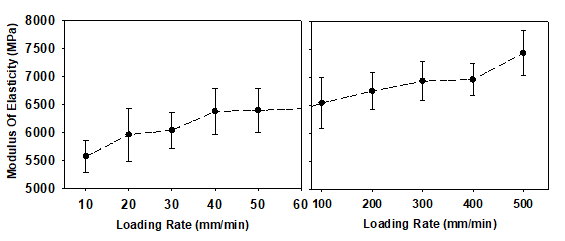Fig. 8. Diagram of the dependence of the modulus of elasticity (E) on the loading rate under compressive force action in the longitudinal direction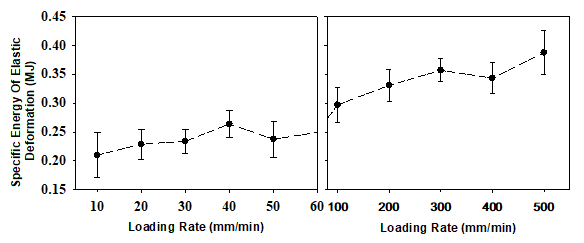Fig. 9. Diagram of the dependence of the specific energy of elastic deformation (eel) on the loading rate under compressive force action in the longitudinal direction

In the study by Fajdig et al. (2016), the modulus of elasticity of beech wood under a compressive action of force in the longitudinal direction is slightly less than 8 GPa at a moisture content (u) of approximately 8%, which corresponds to the values of modulus of elasticity in our study for beech wood samples subjected to loading rate of 500 mm/min.

Results of Measuring the Plastic Properties of Beech Wood

Figures 10 and 11 show the dependences of the mean value of the maximum true stress and the corresponding true strain on the loading rate, respectively. The mean value of the maximum true stress shows an increasing trend as the loading rate increases. The maximum true stress at a loading rate of 500 mm/min is 37.4% higher than the maximum true stress at a loading rate of 10 mm/min, while the corresponding mean value of the maximum true strain does not show a clear dependence on the loading rate.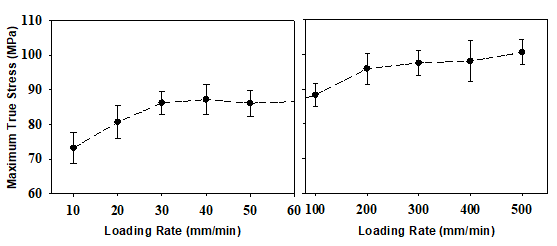Fig. 10. Diagram of the dependence of the maximum true stress (σTmax) on the loading rate under compressive force action in the longitudinal direction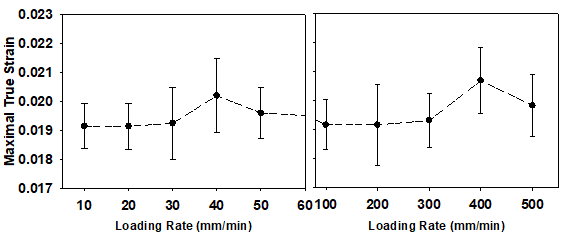Fig. 11. Diagram of the dependence of the maximum true strain (εTmax) on the loading rate under compressive force action in the longitudinal direction

In the study by Koczan and Kozakiewicz (2016), it can be found that the maximum stress of beech wood under compressive force action in the longitudinal direction is approximately 67 MPa at a moisture content (u) of approximately 9%, which is less than the determined values in our experiment. The reason could be the difference in properties of wood beech samples. Moreover, the loading rate could also be the source of variance.

Figures 12 and 13 show the dependence of the mean value of the mean tangent modulus and the specific energy of plastic strain on the loading rate, respectively. The mean value of the tangent modulus and specific energy of plastic strain do not show a clear tendency in the observed loading rate range. The mean value of the tangent modulus at a loading rate of 500 mm/min is 9% higher than the modulus at a loading rate of 10 mm/min, while the mean value of the specific energy of plastic strain at the same loading rate range increased by 30.1%.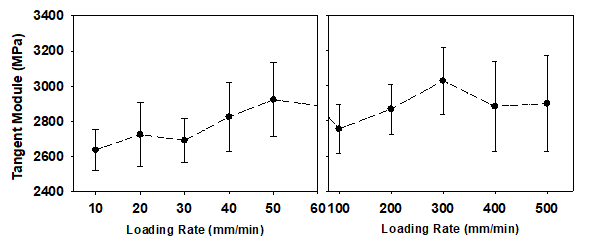Fig. 12. Diagram of the dependence of the tangent module (K) on the loading rate under compressive force action in the longitudinal direction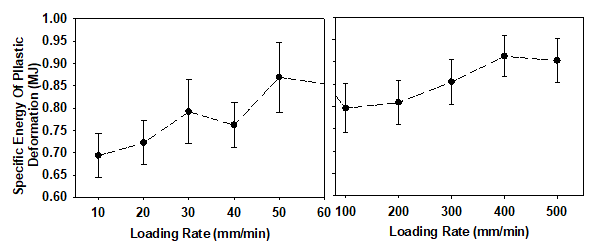Fig. 13. Diagram of the dependence of the specific energy of plastic deformation (epl) on the loading rate under compressive force action in the longitudinal direction

CONCLUSIONS

1. The measured compressive properties of beech wood samples depend on the loading rate in the range of 10 mm/min to 500 mm/min.
2. Increasing the loading rates causes an increase in the measured elastic properties of beech wood. When the loading rate is increased, the mean value of the elastic stresses shows a larger change than the mean values of the elastic strain. Consequently, the result is higher modulus of elasticity with increasing loading rate.
3. Increasing the loading rate increases the mean value of the maximum stress. However, there is no clear trend of changes of the other analysed plastic properties.
4. The standard deviations of the measured plastic properties were drastically larger than the standard deviations of the measured elastic properties, which indicated greater scatter of the measured results in the plastic area of the stress-strain diagram.
5. The presented results showed that wood tends to be more rigid when the compressive forces act quickly. This fact should be taken in account when projecting technology for producing bent curved elements of beech wood for furniture or other products.

REFERENCES CITED

Büyüksarı, Ü. (2017). “Effect of loading rate on mechanical properties of micro-size Scots pine wood,”BioResources 12(2), 2721-2730. DOI: 10.15376/biores.12.2.2721-2730

DIN EN 408: 2012-10 (2012). “Timber structures – Structural timber and glued laminated timber – Determination of some physical and mechanical properties,” Deutsches Institut für Normung, Berlin, Germany.

Fajdig, G., Zafošnik, B., Gospodarič, B., and Straže, A. (2016).”Compression test of thermally-treated beech wood: Experimental and numerical analysis,”BioResources 11(1), 223-234. DOI: 10.15376/biores.11.1.223-234

Horvat, I., and Krpan, J. (1967). Drvnoindustrijski Priručnik [Wood – Processing Manual], Tehnička knjiga Zagreb, Zagreb, Croatia.

Hu, W., Wan, H., and Guan, H. (2019). “Size effect on the elastic mechanical properties of beech and its application in finite element analysis of wood structures,” Forests 10(9), 783-796. DOI: 10.3390/f10090783

Koczan, G., and Kozakiewicz, P. (2016). “Comparative analysis of compression and buckling of European beechwood (Fagus sylvatica L.)” Annals of Warsaw University of Life Sciences – SGGW. Forestry and Wood Technology 95, 81-90.

Mathew, P., and Oxley, P. L. B. (1982). “Predicting the effects of very high cutting speeds on cutting forces,” CIRP Annals 31(1), 49-52. DOI: 10.1016/S0007-8506(07)63267-3

Susainathan, J., Eyma, F., Luycker, E. D., Cantarel, A., Bouvet, C., and Castanie, B. (2019). “Experimental investigation of compression and compression after impact of wood-based sandwich structures,” Composite Structure 2019, 220, 236–249. DOI: 10.1016/j.compstruct.2019.03.095

Tamrakar, S., and Lopez-Anido, R. A. (2010). “Effect of strain rate on flexural properties of wood plastic composite sheet pile” Forest Products Journal 60(5), 465-472. DOI: 10.13073/0015-7473-60.5.465

Wouts, J., Haugou, G., Oudjene, M., Coutellier, D., and Morvan, H. (2016).” Strain rate effects on the compressive response of wood and energy absorption capabilities,” Composite Structure 149, 315-328. DOI: 10.1016/j.compstruct.2016.03.058

Article submitted: November 17, 2020; Peer review completed: January 24, 2021; Revised version received: March 8, 2021; Accepted: March 9, 2021; Published: April 26, 2021.

DOI: 10.15376/biores.16.2.4093-4105